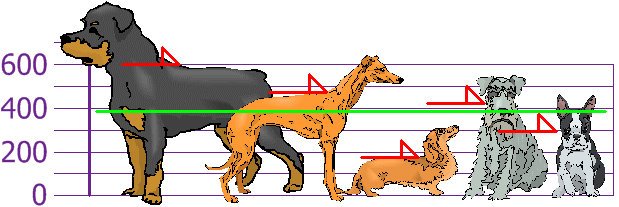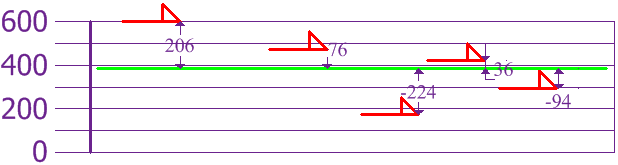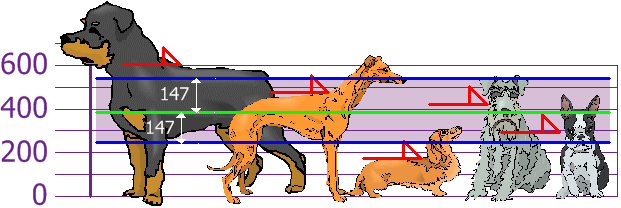# Variance and Standard Deviation

Published:

This lesson covers Variance and Standard Deviation.

Sources:

• https://www.mathsisfun.com
• https://www150.statcan.gc.ca/n1/edu/power-pouvoir/ch12/5214891-eng.htm

# Standard Deviation

• measure of how spread out numbers are

• Single number in comparison to 5-number summary

• square root of the Variance

• $S = \sqrt{Var}$
• Variance is average of the squared differences from the Mean

• $Var = S^2 = \frac{\Sigma(x_i - \bar{x})^2}{n}$
• $Var = S^2 = \frac{\Sigma f(x_i - \bar{x})^2}{\Sigma f}$
• Influenced by outliers

• SD is a good indicator of presence of Outliers
• Standard deviation is also useful when comparing the spread of two separate data sets that have approximately the same mean.

• The data set with the smaller standard deviation has a narrower spread of measurements around the mean and therefore usually has comparatively fewer high or low values.
• An item selected at random from a data set whose standard deviation is low has a better chance of being close to the mean than an item from a data set whose standard deviation is higher.

## Example

• Q: Thirty farmers were asked how many farm workers they hire during a typical harvest season. Their responses were: $4, 5, 6, 5, 3, 2, 8, 0, 4, 6, 7, 8, 4, 5, 7, 9, 8, 6, 7, 5, 5, 4, 2, 1, 9, 3, 3, 4, 6, 4$

• Ans

• Create Frequency Table (may use tally mark to count frequency, $\bcancel{IIII}$)

• xTallyf
0$I$1
1$I$1
2$II$2
3$III$3
4$\bcancel{IIII}I$6
5$\bcancel{IIII}$5
6$IIII$4
7$III$3
8$III$3
9$II$2

$\bar{x} = \frac{\Sigma xf}{\Sigma f} = 5$

• $S = \sqrt{\frac{\Sigma f(x-\bar{x})^2}{\Sigma f}} = 2.25$

## Example

• 220 students were asked the number of hours per week they spent watching television. With this information, calculate the mean and standard deviation of hours spent watching television by the 220 students.

• HoursNumber of students
10 to 142
15 to 1912
20 to 2423
25 to 2960
30 to 3477
35 to 3938
40 to 448

First, using the number of students as the frequency, find the midpoint of time intervals. Now calculate the mean using the midpoint (x) and the frequency (f).

• Ans
• Group $10-14$ represents $9.5 - 14.499$, Similarly $15-19$ represents $14.5 - 19.499$
• Length of Interval is 5; Mid point = 12

## Example

•• Heights: 600mm, 470mm, 170mm, 430mm and 300mm
• Compute Mean, the Variance, and the Standard Deviation
• Mean
• 394
•• Variance
• Each Dog’s Difference from the mean
•• 21704
• Standard Deviation
• 147.32
•• SD is useful since we can show which heights are within one Standard Deviation (147) of the mean (394 mm)
• Using Standard Deviation, we have a standard way of knowing what is normal and what is extra large, or extra small

## Correction for Sample Data

• If the data is population, then variance is average of squared differences
• If the data is sample from a bigger population, we divide by N-1 for calculating variance
• Sample Variance: 27130
• Sample Standard Deviation: 165

## Normal Distribution## Example

• 95% of students are between 1.1m and 1.7m tall. Assume data is normally distributed, compute mean and standard deviation
• Mean is halfway between 1.1m and 1.7m
• Mean = (1.1m + 1.7m) / 2 = 1.4m
• 95% is 2 standard deviations either side of the mean (a total of 4 standard deviations) so:
• $1~SD = \frac{1.7m-1.1m}{4}=0.15$
•It is good to know the standard deviation, because we can say that any value is:

• likely to be within 1 standard deviation (68 out of 100 should be)
• very likely to be within 2 standard deviations (95 out of 100 should be)
• almost certainly within 3 standard deviations (997 out of 1000 should be)

## Properties of standard deviation

• Standard deviation is only used to measure spread or dispersion around the mean of a data set.
• Standard deviation is never negative.
• Standard deviation is sensitive to outliers. A single outlier can raise the standard deviation and in turn, distort the picture of spread.
• For data with approximately the same mean, the greater the spread, the greater the standard deviation.
• If all values of a data set are the same, the standard deviation is zero (because each value is equal to the mean).

When analysing normally distributed data, standard deviation can be used in conjunction with the mean in order to calculate data intervals.

If= mean, S = standard deviation and x = a value in the data set, then

• about 68% of the data lie in the interval
• $\bar{x}-S < x < \bar{x} + S$
• about 95% of the data lie in the interval
• $\bar{x}-2S < x < \bar{x} + 2S$
• about 99% of the data lie in the interval
• $\bar{x}-3S < x < \bar{x}+3S$

Tags: